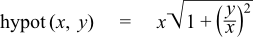#Boost C++ Libraries

...one of the most highly regarded and expertly designed C++ library projects in the world.

This is the documentation for an old version of boost. Click here for the latest Boost documentation.

#### hypot

```template <class T1, class T2>
calculated-result-type hypot(T1 x, T2 y);

template <class T1, class T2, class Policy>
calculated-result-type hypot(T1 x, T2 y, const Policy&);
```

Effects: computesin such a way as to avoid undue underflow and overflow.

The return type of this function is computed using the result type calculation rules when T1 and T2 are of different types.

The final Policy argument is optional and can be used to control the behaviour of the function: how it handles errors, what level of precision to use etc. Refer to the policy documentation for more details.

When calculatingit's quite easy for the intermediate terms to either overflow or underflow, even though the result is in fact perfectly representable.

##### Implementation

The function is even and symmetric in x and y, so first take assume x,y > 0 and x > y (we can permute the arguments if this is not the case).

Then if x * ε   >= y we can simply return x.

Otherwise the result is given by:Copyright © 2006-2010 John Maddock, Paul A. Bristow, Hubert Holin, Xiaogang Zhang, Bruno Lalande, Johan Råde, Gautam Sewani and Thijs van den Berg Distributed under the Boost Software License, Version 1.0. (See accompanying file LICENSE_1_0.txt or copy at http://www.boost.org/LICENSE_1_0.txt)